## Click here to purchase the entire book in PDF format.

5Let’s just chat for a minute why it’s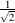. Look back at Figure 1.7 and imagine that the wheel has rotated 45, then draw a right triangle where the hypotenuse is a line connecting the centre of the wheel with the dot on the edge, one side is a line dropping straight down from the dot, and the third side is a horizontal line extending to the right from the centre. Since the angle of rotation is 45, the triangle is isoceles, meaning that the two short sides are of equal length. We also know from Section 1.2 that a =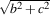. Since b = c, then a =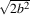. As we said a couple of paragraphs earlier, the radius of the circle (and therefore the length of the hypotenuse) is 1. Therefore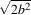= 1. Therefore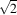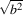= 1. Therefore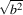= 1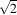. Therefore b = 1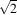.